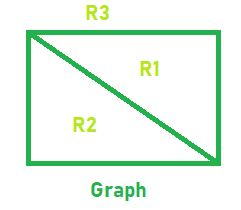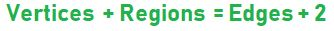Open in App
Not now

# Program to find the number of region in Planar Graph

• Difficulty Level : Basic
• Last Updated : 07 Jun, 2022

Given two integers V and E which represent the number of Vertices and Edges of a Planar Graph. The Task is to find the number of regions of that planar graph.

Planar Graph: A planar graph is one in which no edges cross each other or a graph that can be drawn on a plane without edges crossing is called planar graph.

Region: When a planar graph is drawn without edges crossing, the edges and vertices of the graph divide the plane into regions.

Examples:

Input: V = 4, E = 5
Output: R = 3Input: V = 3, E = 3
Output: R = 2

Approach: Euler found out the number of regions in a planar graph as a function of the number of vertices and number of edges in the graph i.e.Below is the implementation of the above approach:

## C++

 `// C++ implementation of the approach` `#include ` `using` `namespace` `std;`   `// Function to return the number` `// of regions in a Planar Graph` `int` `Regions(``int` `Vertices, ``int` `Edges)` `{` `    ``int` `R = Edges + 2 - Vertices;`   `    ``return` `R;` `}`   `// Driver code` `int` `main()` `{` `    ``int` `V = 5, E = 7;`   `    ``cout << Regions(V, E);`   `    ``return` `0;` `}`

## Java

 `// Java implementation of the approach` `import` `java.io.*;`   `class` `GFG {`   `    ``// Function to return the number` `    ``// of regions in a Planar Graph` `    ``static` `int` `Regions(``int` `Vertices, ``int` `Edges)` `    ``{` `        ``int` `R = Edges + ``2` `- Vertices;`   `        ``return` `R;` `    ``}`   `    ``// Driver code` `    ``public` `static` `void` `main(String[] args)` `    ``{`   `        ``int` `V = ``5``, E = ``7``;` `        ``System.out.println(Regions(V, E));` `    ``}` `}`   `// This code is contributed by akt_mit`

## Python3

 `# Python3 implementation of the approach `   `# Function to return the number ` `# of regions in a Planar Graph ` `def` `Regions(Vertices, Edges) : `   `    ``R ``=` `Edges ``+` `2` `-` `Vertices; `   `    ``return` `R; `   `# Driver code ` `if` `__name__ ``=``=` `"__main__"` `: `   `    ``V ``=` `5``; E ``=` `7``; `   `    ``print``(Regions(V, E)); `   `# This code is contributed ` `# by AnkitRai01`

## C#

 `// C# implementation of the approach` `using` `System;`   `class` `GFG {`   `    ``// Function to return the number` `    ``// of regions in a Planar Graph` `    ``static` `int` `Regions(``int` `Vertices, ``int` `Edges)` `    ``{` `        ``int` `R = Edges + 2 - Vertices;`   `        ``return` `R;` `    ``}`   `    ``// Driver code` `    ``static` `public` `void` `Main()` `    ``{`   `        ``int` `V = 5, E = 7;` `        ``Console.WriteLine(Regions(V, E));` `    ``}` `}`   `// This code is contributed by ajit`

## PHP

 ``

## Javascript

 ``

Output:

`4`

Time Complexity: O(1)

Auxiliary Space: O(1)

My Personal Notes arrow_drop_up
Related Articles